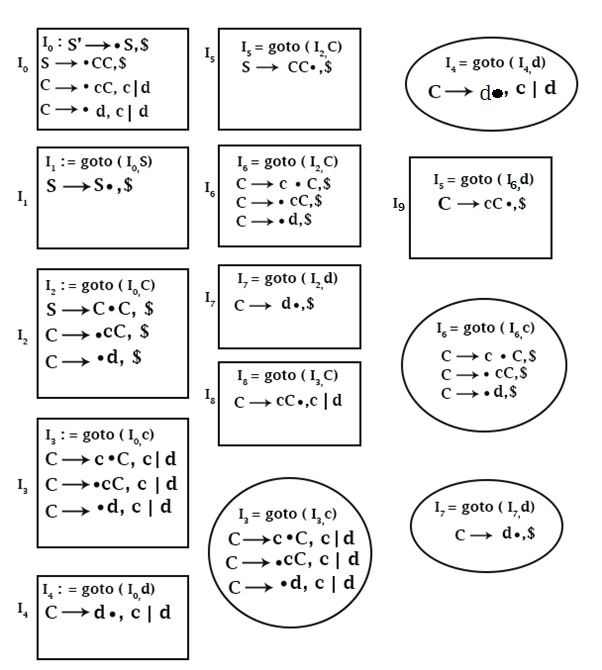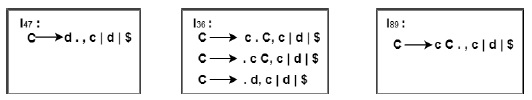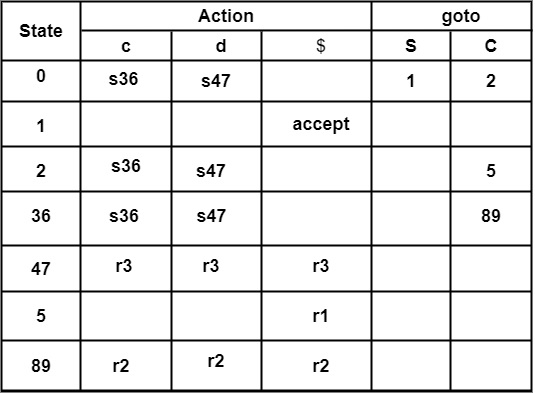# Consider the GrammarnS ‚Üí CCnC ‚Üí c C | dnConstruct the parsing table for LALR (1) parser.

## Solution

Step1 − Construct LR (1) Set of items. First of all, all the LR (1) set of items should be generated.In these states, states I3 and I6 can be merged because they have the same core or first component but a different second component of Look Ahead.

Similarly, states I4 and I7 are the same.

Similarly, states I8 and I9 are the same.

So, I3 and I6 can be combined to make I36.

I4 and I7 combined to make I47.

I8 and I9 combined to make I89.

So, the states will be∴ I3 = goto (I0, c)

But I3 , I6 combined to make I36

∴ I36 = goto (I0, c)

∴ I4 = goto (I0, d)

But I4 , I7 combined to make I47

∴ I47 = goto (I0, d)

∴ I6 = goto (I2, c)

∴ I36 = goto (I2, c)

∴ I7 = goto (I2, d)

∴ I47 = goto (I2, d)

∴ goto (I3, C) = I8

But I8 is now part of I89

∴ goto (I36, C) = I89

Similarly,goto (I3, d) = I4, goto (I6, d) = I7 ∴ goto (I36, d) = I47

Construction of LALR Parsing Table

Filling of "ùê¨ùê°ùê¢ùêüùê≠" Entries(s)

Consider goto(I0, c) = I36

∴ Action[0, c] = s36

∴ Write s36 in front of Row state 0 and column c.

Similarly, consider

goto(I2, d) = I47

∴ Action[2, d] = 47

∴ Write s47 in front of Row State 2 and column d.

Filling the "ùê´ùêûùêùùêÆùêúùêû" Entries (r)

Consider productions of the form A → α ‚àô ,

For example, Consider State

I47 = goto(I0, d)

C → d ‚àô, c |d |$∴ C → d ‚àô, c |d |$ is of form A → α ‚àô , a.

Since C → d is production number (3) in given Question.

∴ Write r3 in front of Row State 47 and column c, d, $. Because c, d looks ahead symbols in production C → d ‚àô , c | d. Filling of goto Entries It can found out only for Non-Terminal. For example, Consider goto(I0, S) = I1 ∴ goto [0, S] = 1 Filling of "ùêÄùêúùêúùêûùê©ùê≠" Entry Since, S′ → S ‚àô ,$ is in I1

∴ Write accept in front of Row state 1 & column \$.

LALR Parsing table can also be obtained by merging the rows of combined states of CLR parsing, i.e., Merge Row corresponding to 3, 6, then 4, 7 and then 8, 9.

The resulting LALR Parsing table will be −